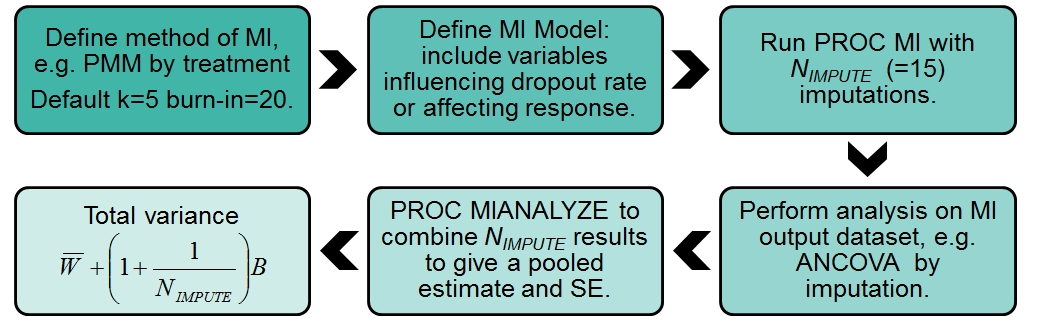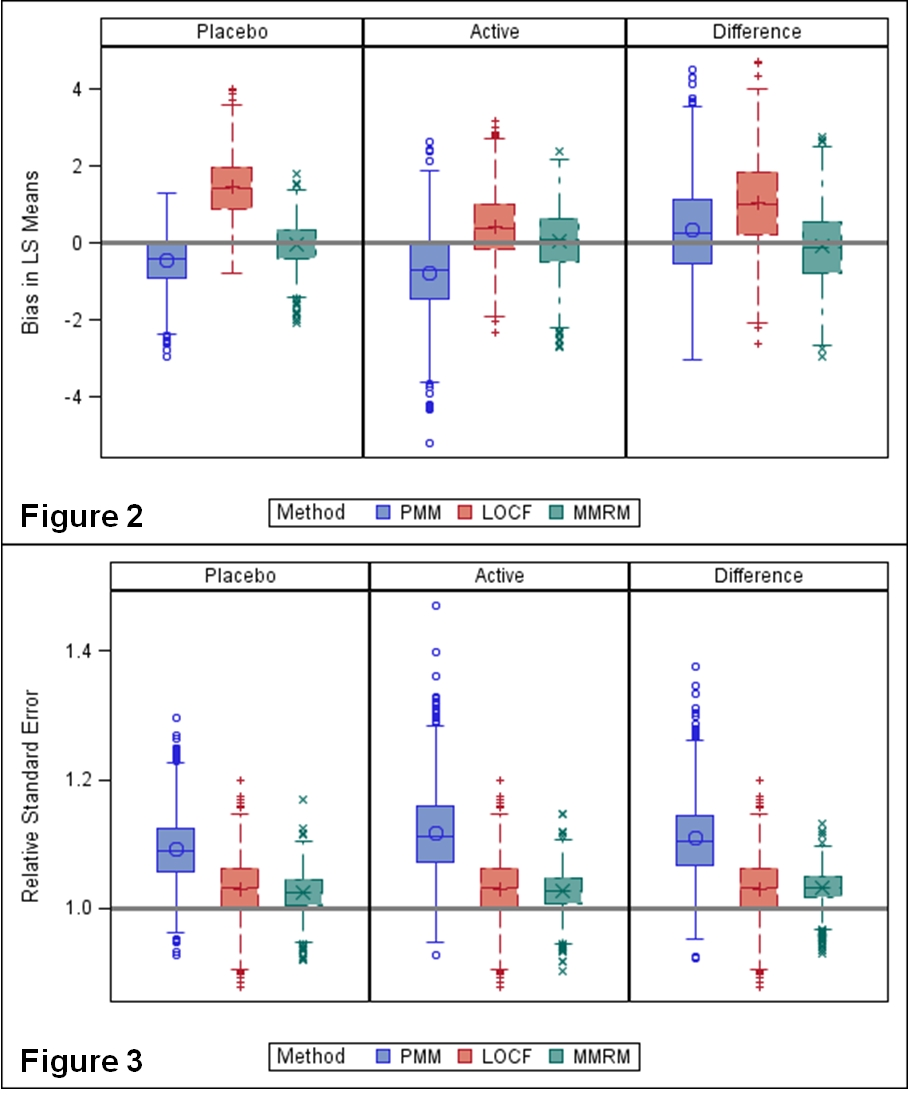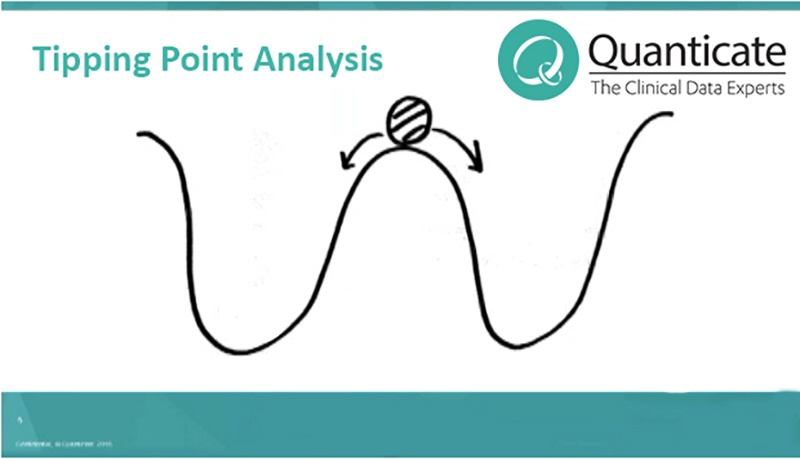<img alt="" src="https://secure.perk0mean.com/171547.png" style="display:none;">

# Multiple Imputation for Handling Missing Data in Clinical Trials### What is Multiple Imputation?

Multiple imputation is a statistical procedure for handling missing data in a study with the aim of reducing the bias, and complications, that missing data can cause. Multiple imputation involves creation of multiple datasets where the missing data are imputed with more realistic values as compared to the non-missing data, allowing for the uncertainty around what the real value might be by imputing data randomly from a distribution. Rubin (1987) developed a method for multiple imputation whereby each of the imputed datasets are analysed, using standard statistical methods, and the results are combined to give an overall result. Analyses based on multiple imputation should then give a result that reflects the true answer while adjusting for the uncertainty of the missing data.

Rubin suggests the following method:

1. Impute the missing values by using an appropriate model which incorporates random variation.
2. Repeat the first step 3-5 times
3. Perform the desired analysis on each data set by using standard, complete data methods.
4. Average the values of the parameter estimates across the missing value samples in order to obtain a single point estimate.
5. Calculate the standard errors by averaging the squared standard errors of the missing value estimates.
6. Calculate the variance of the missing value parameter across the samples.
7. Combine the two quantities in multiple imputation for missing data to calculate the standard errors.

### Multiple Imputation in Clinical Trials

Standard Multiple Imputation (MI) performs the imputations such that the results for the subject with the missing data tend towards the mean for the treatment group they belong to, due to the weakening of the within subject correlation. This also results in an increase in the variance with time, as is expected in clinical trials. The realization that the subjects who withdraw are no longer on randomized treatment, led to developments to allow imputation based on a clinically plausible post-withdrawal path. One of these is multiple imputation under the copy reference (CR) assumption where post-withdrawal data is modeled assuming that the subject was a member of the reference group. Here, the outcome would tend towards the mean for the reference group.

When single imputation is used within clinical trials it is usually done in one of three ways; impute with the mean of all observed data (mean imputation), impute with the last observed value (last observation carried forward) or impute with the worst possible value (worst case imputation). All of these methods have advantages and disadvantages and the most appropriate method to use will depend on the pattern of missing data and the hypothesis being tested

### Case Study on MI

In a case study examined to look at MI in clinical trials, comparing Active to Placebo treatment (at Weeks 2, 4, 6 and 12 of the trial) in adolescents with acne, drop outs were common.  The primary endpoint was the number of lesions at Week 12.  The factors believed to affect the propensity to be missing included age, side effects and lack of efficacy, and thus missing data patterns differ between groups.

It is common for datasets of this type to be analysed using an analysis of covariance (ANCOVA) of last observation carried forward (LOCF) data.  MI methods can be programmed in PROC MI in SAS Version 9.3 offering an alternative method to deal with missing data; we explore the MI process, compare results with LOCF ANCOVA and a mixed models repeated measures (MMRM) and ask is it worth the effort?

### Methodology

A simulation of 1000 data sets was carried out by removing data randomly from a completer dataset (N=131) using propensity scores based on the pattern of missing data observed in the full dataset (N=153).  Least squares (LS) means and differences were estimated with standard errors (SE).  Boxplots are presented of the bias and relative SE from MI compared to LOCF ANCOVA and a MMRM approach without imputation of data; these are relative to the ANCOVA on the completer dataset.

We focus on the least biased of several methods of MI tested: Predictive Mean Matching (PMM) which imputes values by sampling from k observed data points closest to a regression predicted value where the regression parameters are sampled from a posterior distribution.  The total variance of combined ANCOVA results (see Figure 1) is calculated from the average within-imputation (W) and between-imputation variance (B). ,Figure 1:  Flow chart of Multiple Imputation Process

### Results

MMRM is the least biased and LOCF the most biased of the three methods (Figure 2). Relative SEs were largest for PMM (Figure 3).Figure 2: Bias in LS Means of Estimate

Figure 3: Relative standard error of difference in treatment means

Both figures show distribution from 1000 simulations (data were removed randomly based on propensity scores; the propensity model included age, side effect of pain after treatment and efficacy measured by lesion counts). Bias and relative standard errors are relative to the completer dataset.

### Discussion

The Food and Drug Administration (FDA) were critical of the use of LOCF in Phase 3; it assumes no trend of response over time resulting in bias and a distorted covariance structure.  All methods in PROC MI and MMRM make the assumption that data are Missing at Random (MAR).  PROC MI has useful functionality in summarising the missing data patterns.

MI is complex to define a priori as there are many details to consider (see Figure 1) and additional data processing steps are necessary.  The PMM method of imputation has the advantage over alternative MI methods in that no bounds, rounding or post-imputation manipulation is required to give plausible imputed lesion counts.

Sensitivity analyses can investigate a range of delta (δ) values added to imputed values to explore the robustness of conclusions to imputation.

Relative SEs were generally greater than 1 for all methods, this is to be expected given the loss of approximately 15% of data from the completer dataset by using the propensity scores in the simulation of missing values.  The SEs from MI techniques incorporate an additional component (B) to account for the uncertainty in the imputation, whereas LOCF ignores this uncertainty.  However, the resulting SE from MI is appreciably larger than that from MMRM, and thus this MI method has less power.

### Conclusion

MI is complex to define and computationally intensive and thus would need to have substantial benefits to be worth the effort for a primary analysis.  We found PMM to have less power than MMRM without reducing bias.  Therefore, we recommend: MMRM as the primary analysis; use of PROC MI to investigate the sensitivity (delta method); and avoiding LOCF.  Further work could investigate scenarios such as data not being MAR, varying k and whether the default burn-in of 20 in PMM is sufficient.Related Blogs

References

 SAS/STAT(R) 12.1 User's Guide, "The MIANALYZE Procedure, Combining Inferences from Imputed Data Sets," [Online]. Available:

 D. Rubin, Multiple Imputation for Nonresponse in Surveys, New York: John Wiley & Sons, 1987.

This article was featured as a poster at the PSI 2014 annual conference and was published on 30/06/2014. It has since been updated.

## Related Articles#### [Video] Tipping Point Analysis in Multiple Imputation for Binary Missing Data

by Statistical Consultancy Team#### An Introduction to Estimands [Podcast]

by Statistical Consultancy Team